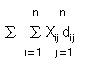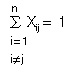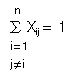# 110.C++ Neural Networks and Fuzzy Logic by Valluru B. Rao M&T Books, IDG Books Worldwide, Inc. ISBN: 1558515526   Pub Date: 06/01/95

### Traveling Salesperson Problem

The traveling salesperson problem is well-known in optimization. Its mathematical formulation is simple, and one can state a simple solution strategy also. Such a strategy is often impractical, and as yet, there is no efficient algorithm for this problem that consistently works in all instances. The traveling salesperson problem is one among the so- called NP-complete problems, about which you will read more in what follows. That means that any algorithm for this problem is going to be impractical with certain examples. The neural network approach tends to give solutions with less computing time than other available algorithms for use on a digital computer. The problem is defined as follows. A traveling salesperson has a number of cities to visit. The sequence in which the salesperson visits different cities is called a tour. A tour should be such that every city on the list is visited once and only once, except that he returns to the city from which he starts. The goal is to find a tour that minimizes the total distance the salesperson travels, among all the tours that satisfy this criterion.

A simple strategy for this problem is to enumerate all feasible tours—a tour is feasible if it satisfies the criterion that every city is visited but once—to calculate the total distance for each tour, and to pick the tour with the smallest total distance. This simple strategy becomes impractical if the number of cities is large. For example, if there are 10 cities for the traveling salesperson to visit (not counting home), there are 10! = 3,628,800 possible tours, where 10! denotes the factorial of 10—the product of all the integers from 1 to 10—and is the number of distinct permutations of the 10 cities. This number grows to over 6.2 billion with only 13 cities in the tour, and to over a trillion with 15 cities.

#### The TSP in a Nutshell

For n cities to visit, let Xij be the variable that has value 1 if the salesperson goes from city i to city j and value 0 if the salesperson does not go from city i to city j. Let dij be the distance from city i to city j. The traveling salesperson problem (TSP) is stated as follows:

Minimize the linear objective function:subject to:for each j=1, …, n (linear constraint)for each i=1, …, n (linear constraint)
ɷXij for 1 for all i and j (integer constraint)

This is a 0-1 integer linear programming problem.

#### Solution via Neural Network

This section shows how the linear and integer constraints of the TSP are absorbed into an objective function that is nonlinear for solution via Neural network.

The first consideration in the formulation of an optimization problem is the identification of the underlying variables and the type of values they can have. In a traveling salesperson problem, each city has to be visited once and only once, except the city started from. Suppose you take it for granted that the last leg of the tour is the travel between the last city visited and the city from which the tour starts, so that this part of the tour need not be explicitly included in the formulation. Then with n cities to be visited, the only information needed for any city is the position of that city in the order of visiting cities in the tour. This suggests that an ordered n-tuple is associated with each city with some element equal to 1, and the rest of the n – 1 elements equal to 0. In a neural network representation, this requires n neurons associated with one city. Only one of these n neurons corresponding to the position of the city, in the order of cities in the tour, fires or has output 1. Since there are n cities to be visited, you need n2 neurons in the network. If these neurons are all arranged in a square array, you need a single 1 in each row and in each column of this array to indicate that each city is visited but only once.

Let xij be the variable to denote the fact that city i is the jth city visited in a tour. Then xij is the output of the jth neuron in the array of neurons corresponding to the ith city. You have n2 such variables, and their values are binary, 0 or 1. In addition, only n of these variables should have value 1 in the solution. Furthermore, exactly one of the x’s with the same first subscript (value of i) should have value 1. It is because a given city can occupy only one position in the order of the tour. Similarly, exactly one of the x’s with the same second subscript (value of j) should have value 1. It is because a given position in the tour can be only occupied by one city. These are the constraints in the problem. How do you then describe the tour? We take as the starting city for the tour to be city 1 in the array of cities. A tour can be given by the sequence 1, a, b, c, …, q, indicating that the cities visited in the tour in order starting at 1 are, a, b, c, …, q and back to 1. Note that the sequence of subscripts a, b, …, q is a permutation of 2, 3, … n – 1, xa1=1, xb2=1, etc.

Having frozen city 1 as the first city of the tour, and noting that distances are symmetric, the distinct number of tours that satisfy the constraints is not n!, when there are n cities in the tour as given earlier. It is much less, namely, n!/2n. Thus when n is 10, the number of distinct feasible tours is 10!/20, which is 181,440. If n is 15, it is still over 43 billion, and it exceeds a trillion with 17 cities in the tour. Yet for practical purposes there is not much comfort knowing that for the case of 13 cities, 13! is over 6.2 billion and 13!/26 is only 239.5 million—it is still a tough combinatorial problem.C++ Neural Networks and Fuzzy Logic
ISBN: 1558515526
EAN: 2147483647
Year: 1995
Pages: 139

Similar book on Amazon